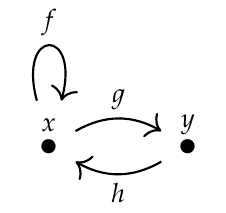Robert wrote:

> Who would have guessed: glancing at a problem and putting down one's first hunch unchecked tends to give wrong answers :-D

Who would have guessed! It's fine to make guesses here; I'm more interested in getting people thinking - and thinking out loud - than having people be infallible.

I believe your original formula was correct except that you need to count _ordered_ sums of the loop lengths \\(a_i\\), not ordinary sums. For example, the Fibonacci graph has loops of length 1 and 2 based at the point \\(x\\):and the number of loops of length \\(n\\) based at this point is the number of ways of writing \\(n\\) as an _ordered_ sums of 1's and 2's. For example

\[ 4 = 1 + 1 + 1 + 1 = 2 + 1 + 1 = 1 + 2 + 1 = 1 + 1 + 2 = 2 + 2 \]

so there are four loops of length 5... and the fourth Fibonacci number is 5.